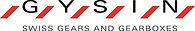top of page

Conversion Factors:

1 Newton Meter (Nm) = 1000 mN-m = 141.6119 Oz-In = 8.850746 Lb-In

1 Ounce Inch (Oz-In) = 0.0070615 Nm = 7.0615 mN-m

1 Pound Inch (Lb-In) = 16 Oz-In = 0.1129848 Nm = 112.9848 mN-m

1 Inch = 2.54 Centimeters (cm) = 25.4 Millimeters (mm)

10 Millimeters (mm) = 1 Centimeter (cm) = 0.3937 Inches (In)bottom of page